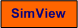# Serial and Parallel PID Controller

Snapshot of a part of the front panel of the simulator: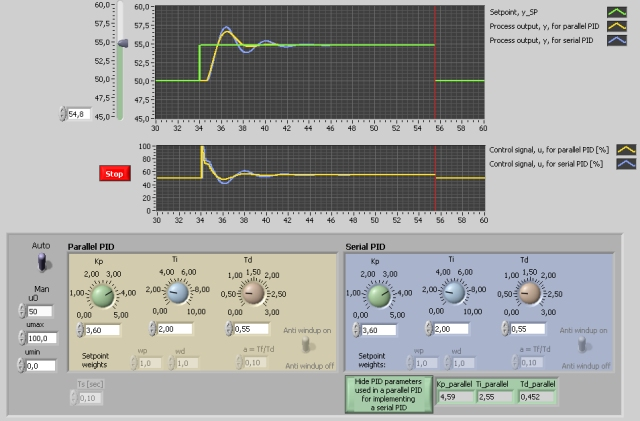• What is needed to run the the simulator? Read to get most recent information!
• Tips for using the simulator.
• The simulator: . The simulator runs immediately after the download by clicking Open in the download window. Alternatively, you can first save a copy of the exe-file on any directory (folder) on your PC and then run the exe-file, which starts the simulator.

## Description of the system to be simulated

Some commercial PID controllers implement a PID controller function on serial form, while others use the parallel form. The serial form corresponds closer to the former pneumatic controllers. In this simulator two PID control systems are simulated simultaneously - one with a serial PID controller and one with a parallel PID controller. Therefore, it is easy to compare the behaviour of these two control systems. The process which is controlled is given by a transfer function model, namely a second order system in series with a transport delay.

In the simulator the user can choose to transform the PID parameters for a serial PID controller to parameters for a parallel controller so that the parallel controller behaves like a serial controller.

Here is the parallell PID controller: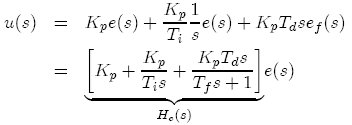Here is the serial PID controller: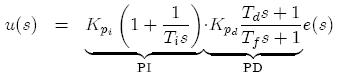Here are the transformations from serial to parallell PID settings: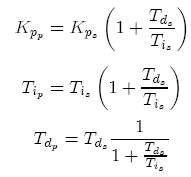## Aim

The aims of this simulator is to observe differences between a PID controller on serial form and a PID controller on parallel form, and to see if there is any use of transforming parallel PID parameters to serial PID parameters.

## Motivation

There are both serial and parallel commercial PID controllers. It is useful to see if there is any substantial difference between these two controllers. Furthermore, it may be useful to see if a transformation from serial PID parameters to parallel PID parameters has any important effect.